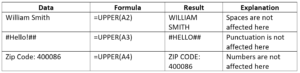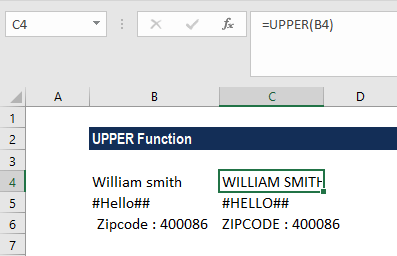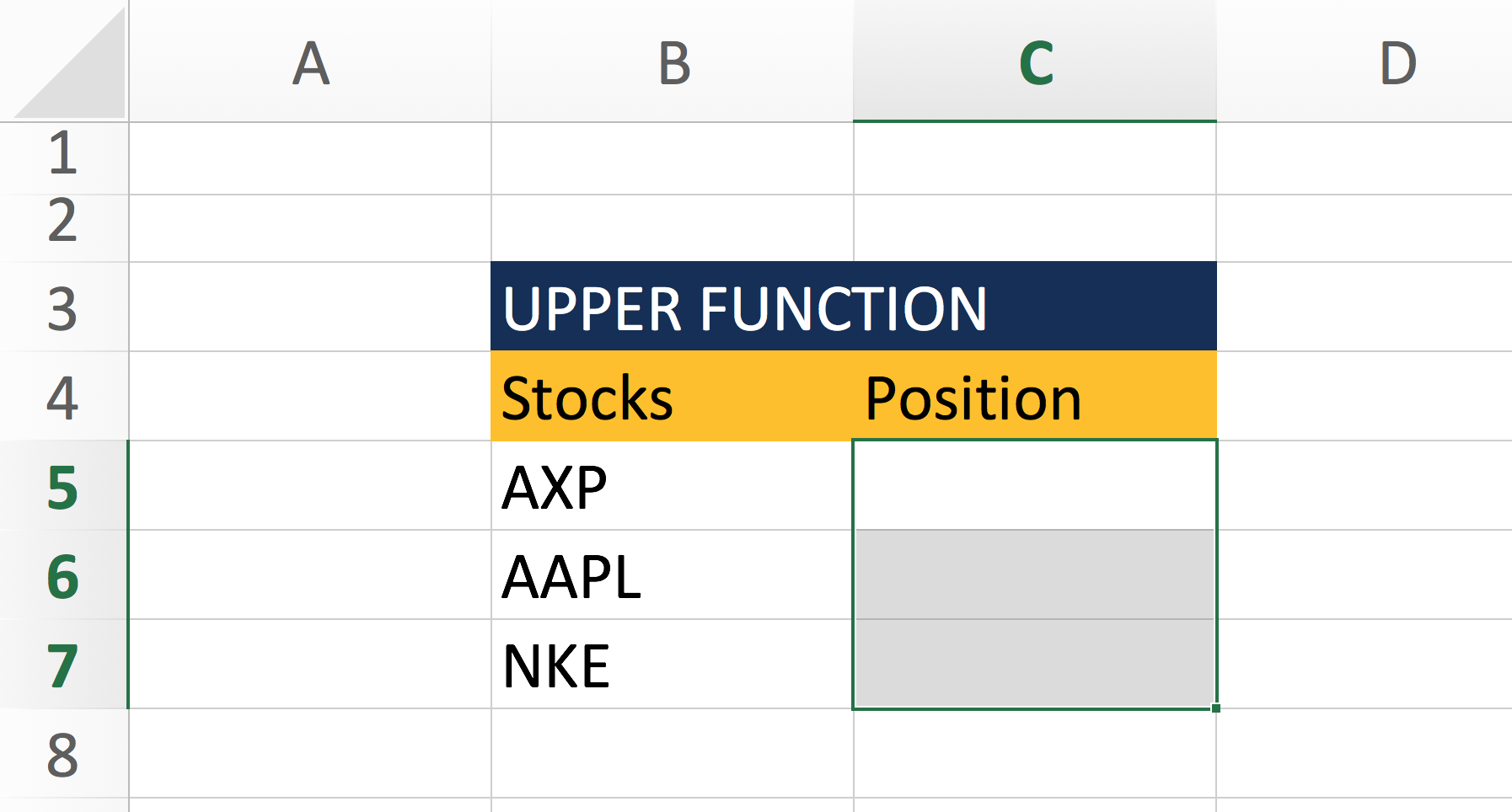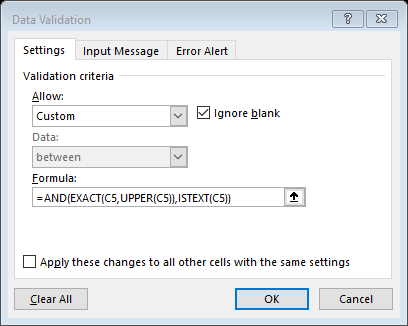# UPPER Function

Converts text to upper case

## UPPER Function – How to convert text to all capital letters?

The UPPER function is an Excel Text function, that will convert text to all capital letters (UPPERCASE). Thus, the function converts all characters in a supplied text string into upper case.

In financial analysis, we often import data from external sources. The UPPER function will help us convert text to upper case if required. It will not affect numbers and punctuations. Cleaning up data for analysis is often a very time-consuming step, Text functions are often great tools to speed up the process.

### Formula

=UPPER(Text)

1. Text (required argument) – This is the text that we want to convert to uppercase. Text can be a text string or a reference to a cell.

### How to use the UPPER Function in Excel?

The UPPER function was introduced in Excel 2007 and is available in all subsequent Excel versions. To understand the uses of the function, let us consider a few examples:

#### Example – How the function works

Let’s see how the function will behave when we give the following formula:We get the results below:As you can see only letters were affected on the string. Numbers and symbols were left unchanged.

#### Example – UPPER Function for Data Validation

Suppose you want to prevent a user from inputting lowercase text. This can be done, by using data validation and entering a customized formula with the UPPER, AND, and EXACT functions. So assume that we start off with the following table, and want to prevent lowercase letters on cells C5:C7We apply data validation to the cells C5:C7, with the following function:

=AND(EXACT(C5, UPPER(C5)),ISTEXT(C5))Data Validation will then prevent users from entering strings that are not upper case text. The function above will check for two things – if the data entered on the selected cells are text AND if the data entered is in UPPER case. Data Validation is always checking the input of the cells, to ensure that only correct data is entered in those cells.

### Things to remember about the UPPER Function

1. All letters in text are converted to uppercase.
2. Numbers and punctuation characters are not affected.

Thanks for reading CFI’s guide to important Excel functions! By taking the time to learn and master these functions, you’ll significantly speed up your financial analysis and valuation modeling. To learn more, check out these additional CFI resources:

• Excel Formulas for Finance